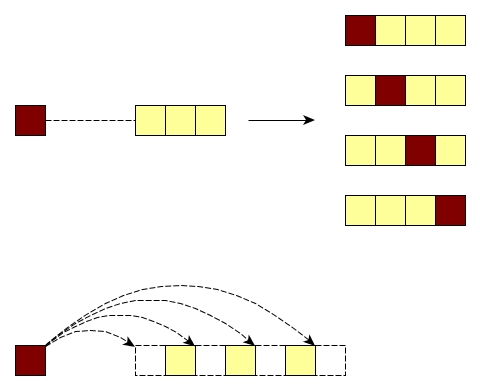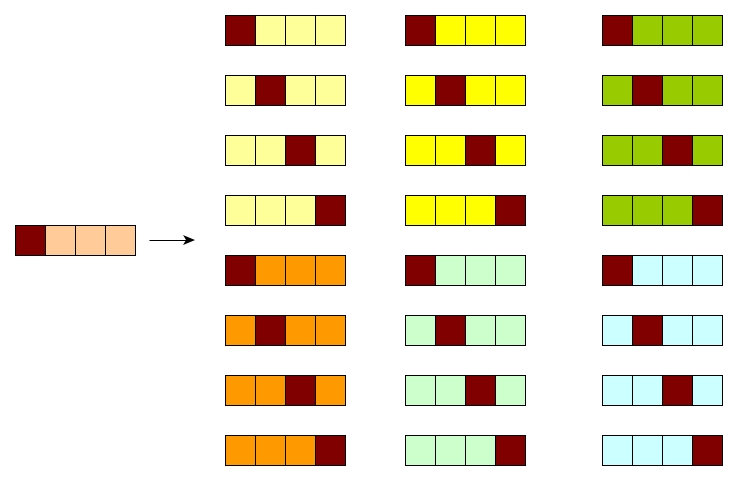# Permutation

Van C. Ngo · November 22, 2016

In this post, I will talk about a common approach for generating all permutations, its complexity, and how big the argument list can be that makes the stack overflow using OCaml. I borrow some pictures and work from typeocaml.

## The insert-into-all-positions solution

Let’s assume that we have a set of all permutations for a set of 3 elements. Thus totally there are 6 permutations. For example, let 3 elements be [1;2;3], then 6 permutations are [1;2;3], [2;1;3], [2;3;1], [1;3;2], [3;1;2], and [3;2;1].

So now what if we put a new element, say 4, into the set of 4 elements [1;2;3;4]? What we need to do is combining the new element 4 into the previous set of permutations to generate a new set of permutations.

Let’s see how a new element is inserted into a permutation. Consider a permutation of 3 elements [1;2;3], there are 4 possible positions (before 1, 2, 3, and after 3) to insert 4 as shown in the figure.Therefore, with 6 previous permutations we have totally $$4 * 6 = 24$$ new permutations if we insert the new element 4.## Code

The implementation can be done by the following two functions. The insert_all_positions function inserts a new element given a set of permutations. The permutations function recursively generates all permuations. If the number of elements is 0 or 1, then there is only 1 permutation. Here is the Gist.

let insert_all_positions x l =
let rec aux prev acc l =
match l with
| [] -> (prev @ [x]) :: acc |> List.rev
| hd::tl as l -> aux (prev @ [hd]) ((prev @ [x] @ l) :: acc) tl
in aux [] [] l;;

let rec permutation l =
match l with
| [] -> []
| hd::[] -> [[hd]]
| hd::tl -> List.fold_left (fun acc p -> acc @ insert_all_positions hd p) [] (permutation tl);;


## Complexity

The complexity depends on the number of permuations which is $$n!$$ (n is the number of elements). Assume that the complexity of insert a new element to a permuation takes 1 time unit, thus for a permuation consisting of m elements, it takes $$(m + 1)$$ time units. Therefore for each recursive call, we have the complexity $$T(m) = m * T(m - 1)$$. The complexity of the algorithm should be

$$T(n) = n * T(n - 1)$$ $$= n * (n - 1) * T(n - 2)$$ $$= ...$$ $$= n * (n-1) * (n - 2) * ... * 2 * T(1) = n!$$

## Stack overflow

When I run the code above with OCaml version 4.02.1, stack overflow occurs likely with the number of elements is 9. For example,

permutation [1;2;3;4;5;6;7;8;9];;
Stack overflow during evaluation (looping recursion?).


## Dynamic permutations

One interesting way to deal with big number of elements is generating one permutation at each time. One can employ the Johnson Trotter algorithm to generate a different permutation each time. I will talk about this algorithm in a next post.